Question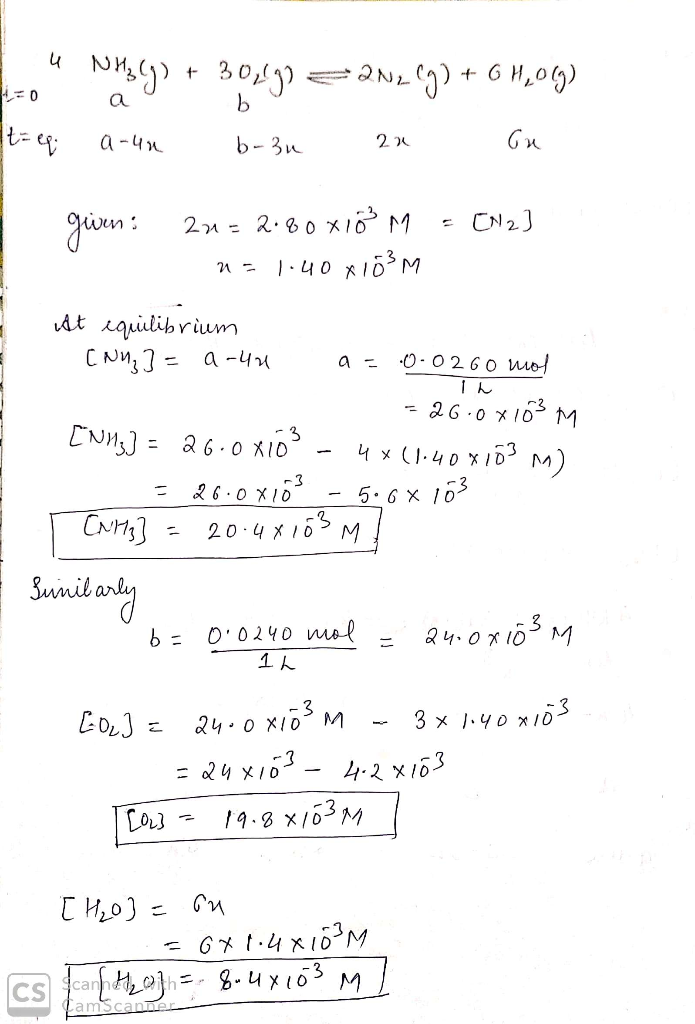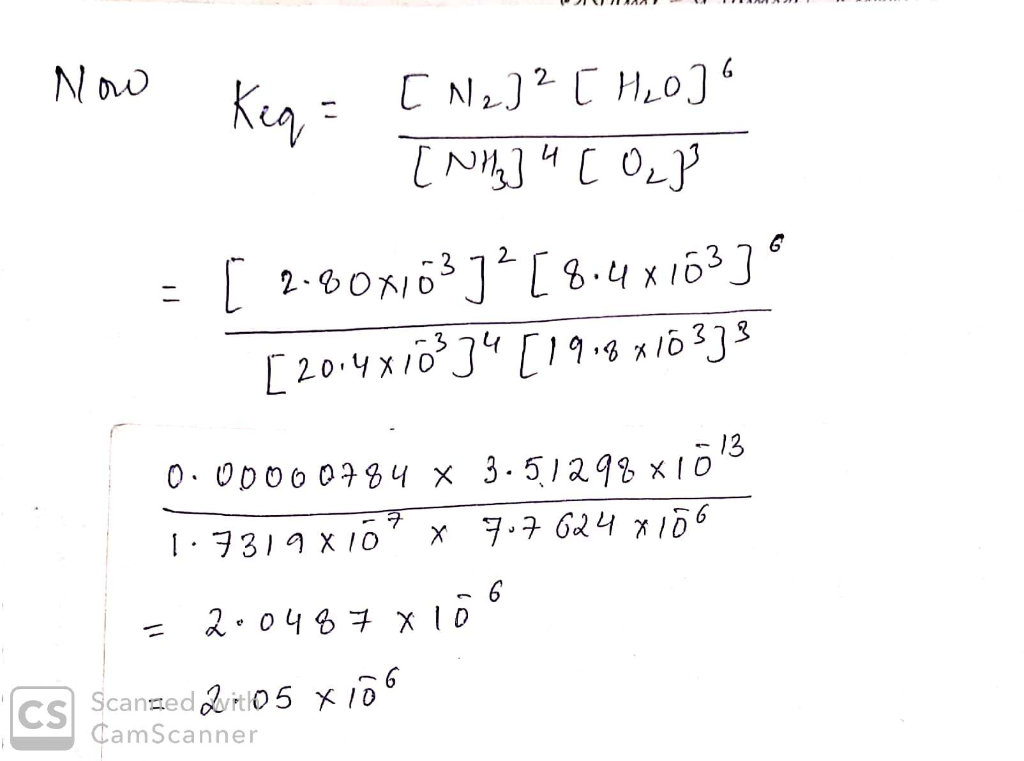#### Earn Coins

Coins can be redeemed for fabulous gifts.

Similar Homework Help Questions
• ### The first step in industrial nitric acid production is the catalyzed oxidation of ammonia. Without a...

The first step in industrial nitric acid production is the catalyzed oxidation of ammonia. Without a catalyst, a different reaction predominates: 4NH3(g) + 3O2(g) ⇔ 2N2(g) + 6H2O(g) When 0.0160 mol gaseous NH3 and 0.0220 mol gaseous O2 are placed in a 1.00 L container at a certain temperature, the N2 concentration at equilibrium is 2.70×10-3 M. Calculate Keq for the reaction at this temperature.

• ### The first step in industrial nitric acid (HNO3) production is the catalyzed oxidation of ammonia (NH3)....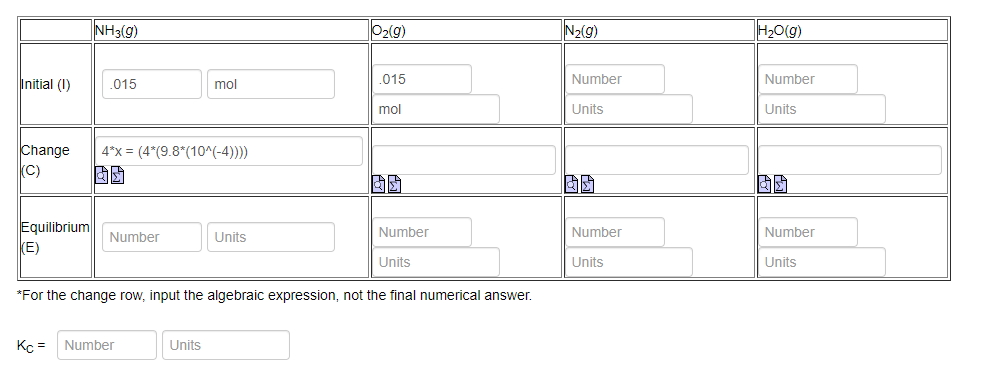The first step in industrial nitric acid (HNO3) production is the catalyzed oxidation of ammonia (NH3). Without the catalyst the following reaction predominates: 4NH3(g) + 3O2(g) ⇔ 2N2(g) + 6H2O(g). When 0.0150 mol each of NH3(g) and O2(g) are placed in a 1.00 L container at a certain temperature the N2(g) concentration at equilibrium is 1.96x10-3 M. Fill in the following ICE table and calculate the resulting KC. NH3(9) 02(9) N2(9) H20(9) Initial (1) 015 mol mol .015 Number Number...

• ### The industrial production of nitric acid (HNO3) is a multistep process. The first step is the oxidation of ammonia (NH...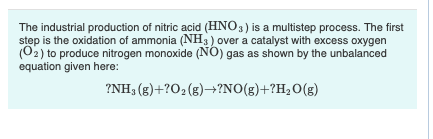The industrial production of nitric acid (HNO3) is a multistep process. The first step is the oxidation of ammonia (NH3 ) over a catalyst with excess oxygen (02) to produce nitrogen monoxide (NO) gas as shown by the unbalanced equation given here: ?NH, (g)+?O2(g) +?NO(g)+?H2O(g) Part A What volume of O2 at 684 mmHg and 39 °C is required to synthesize 19.0 mol of NO? Express your answer to three significant figures and include the appropriate units. View Available Hint(s)...

• ### The industrial production of nitric acid (HNO3) is a multistep process. The first step is the oxi...

The industrial production of nitric acid (HNO3) is a multistep process. The first step is the oxidation of ammonia (NH3) over a catalyst with excess oxygen (O2) to produce nitrogen monoxide (NO) gas as shown by the unbalanced equation given here: NH3(g)+?O2(g)→?NO(g)+?H2O(g) What volume of O2 at 722 mmHg and 27 ∘C is required to synthesize 17.5 mol of NO?

• ### The industrial production of nitric acid (HNO3) is a multistep process. The first step is the...

The industrial production of nitric acid (HNO3) is a multistep process. The first step is the oxidation of ammonia (NH3) over a catalyst with excess oxygen (O2) to produce nitrogen monoxide (NO) gas as shown by the unbalanced equation given here: ?NH3(g)+?O2(g)→?NO(g)+?H2O(g) What volume of O2 at 798 mmHg and 41 ∘C is required to synthesize 12.5 mol of NO? Express your answer to three significant figures and include the appropriate units.

• ### The industrial production of nitric acid (HNO3) is a multistep process. The first step is the...

The industrial production of nitric acid (HNO3) is a multistep process. The first step is the oxidation of ammonia (NH3) over a catalyst with excess oxygen (O2) to produce nitrogen monoxide (NO) gas as shown by the unbalanced equation given here: ?NH3(g)+?O2(g)→?NO(g)+?H2O(g) Part A What volume of O2 at 684 mmHg and 41 ∘C is required to synthesize 19.0 mol of NO? Express your answer to three significant figures and include the appropriate units. volume of O^2=

• ### Nitric acid is produced commercially by a multi-step process, starting from the oxidation of ammonia. If...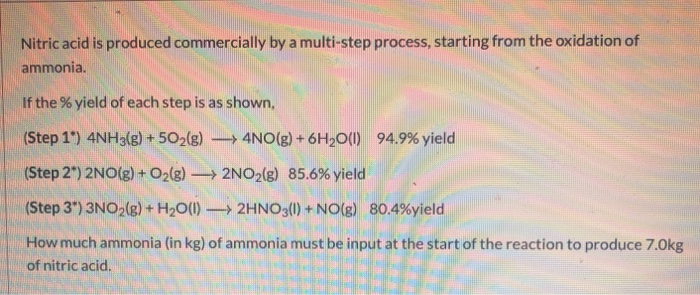Nitric acid is produced commercially by a multi-step process, starting from the oxidation of ammonia. If the % yield of each step is as shown (Step 1.) 4NH3(g) + 502(g)-→ 4N0(g) + 6H20(1) 94.9% yield Step 272NO(g) +02(g) 2NO2(g) 85.6% yield Step 3*) 3NO2(g) + H20(I)-> 2 HNO3(l) + NO(g) 80.4%yield How much ammonia (in kg) of ammonia must be input at the start of the reaction to produce 7.0kg of nitric acid.

• ### Nitric acid is a key industrial chemical, largely used to make fertilizers and explosives. The first...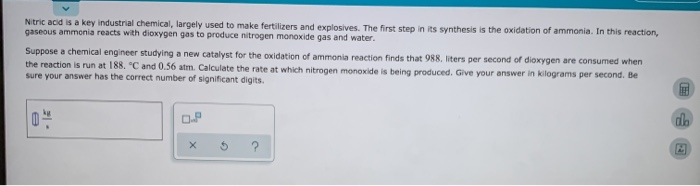Nitric acid is a key industrial chemical, largely used to make fertilizers and explosives. The first step in its synthesis is the oxidation of ammonia. In this reaction, gaseous ammonia reacts with dioxygen gas to produce nitrogen monoxide gas and water. Suppose a chemical engineer studying a new catalyst for the oxidation of ammonia reaction finds that 988 liters per second of dioxygen are consumed when the reaction is run at 188. "C and 0.56 atm. Calculate the rate at...

• ### Nitric acid is a key industrial chemical, largely used to make fertilizers and explosives. The first...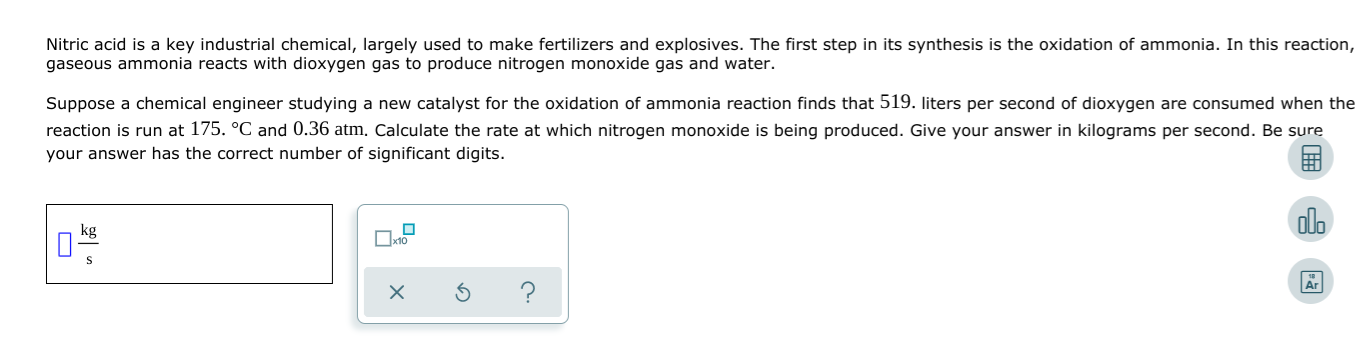Nitric acid is a key industrial chemical, largely used to make fertilizers and explosives. The first step in its synthesis is the oxidation of ammonia. In this reaction, gaseous ammonia reacts with dioxygen gas to produce nitrogen monoxide gas and water. Suppose a chemical engineer studying a new catalyst for the oxidation of ammonia reaction finds that 519. liters per second of dioxygen are consumed when the reaction is run at 175. C and 0.36 atm. Calculate the rate at...

• ### An Important step in the synthesis of nitric acid is the conversion of ammonia to nitric...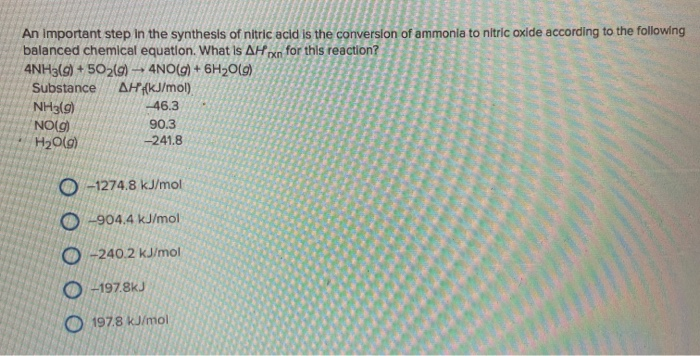An Important step in the synthesis of nitric acid is the conversion of ammonia to nitric oxide according to the following balanced chemical equation. What is AHpxn for this reaction? 4NH3(g) + 5O2(g) -4NO(g) + 6H2O(9) Substance AH?f(kJ/mol) NH3(g) -46.3 NO(9) 90.3 H2O(9) -241.8 0 -1274.8 kJ/mol -904.4 kJ/mol 0 -240.2 kJ/mol 0 -197.8kJ 197.8 kJ/mol